# PyNHD: Navigate and subset NHDPlus database## Features#

PyNHD is a part of HyRiver software stack that is designed to aid in hydroclimate analysis through web services.

These web services can be used to navigate and extract vector data from NHDPlus V2 (both mid- and high-resolution) database such as catchments, HUC8, HUC12, GagesII, flowlines, and water bodies.

Moreover, the PyGeoAPI service provides four functionalities:

1. `flow_trace`: Trace flow from a starting point to up/downstream direction.

2. `split_catchment`: Split the local catchment of a point of interest at the point’s location.

3. `elevation_profile`: Extract elevation profile along a flow path between two points.

4. `cross_section`: Extract cross-section at a point of interest along a flow line.

PyNHD also provides access to the entire NHDPlus dataset for CONUS (L48) via `nhdplus_l48` function. You can get any of the 31 layers that are available in the NHDPlus dataset. You can also get NHDPlus Value Added Attributes on Hydroshare and ENHD. These datasets that do not have geometries, include slope and roughness, among other attributes, for all NHD flowlines. You can use `nhdplus_vaa` and `enhd_attrs` functions to get these datasets.

Additionally, you can get many more derived attributes at NHD catchment-level through two sources:

• Select Attributes for NHDPlus Version 2.1 Reach Catchments from an item on ScienceBase

• EPA’s StreamCat dataset.

They both include hundreds of attributes such as hydroclimate properties, water quality, urbanization, and population. In addition to NHD catchment summaries, they also have their network-accumulated values (both upstream and divergence-routed). You can use `nhdplus_attrs`, `epa_nhd_catchments`, `streamcat` functions to get these datasets.

Additionally, PyNHD offers some extra utilities for processing the NHD flowlines:

• `flowline_xsection` and `network_xsection`: Get cross-section lines along a flowline at a given spacing or a network of flowlines at a given spacing.

• `flowline_resample` and `network_resample`: Resample a flowline or network of flowlines based on a given spacing. This is useful for smoothing jagged flowlines similar to those in the NHDPlus database.

• `prepare_nhdplus`: For cleaning up the data frame by, for example, removing tiny networks, adding a `to_comid` column, and finding terminal flowlines if it doesn’t exist.

• `topoogical_sort`: For sorting the river network topologically which is useful for routing and flow accumulation.

• `vector_accumulation`: For computing flow accumulation in a river network. This function is generic, and any routing method can be plugged in.

These utilities are developed based on an R package called nhdplusTools and a Python package called nldi-xstool.

All functions and classes that request data from web services use `async-retriever` that offers response caching. By default, the expiration time is set to never expire. All these functions and classes have two optional parameters for controlling the cache: `expire_after` and `disable_caching`. You can use `expire_after` to set the expiration time in seconds. If `expire_after` is set to `-1`, the cache will never expire (default). You can use `disable_caching` if you don’t want to use the cached responses. The cached responses are stored in the `./cache/aiohttp_cache.sqlite` file.

You can find some example notebooks here.

Moreover, under the hood, PyNHD uses AsyncRetriever for making requests asynchronously with persistent caching. This improves the reliability and speed of data retrieval significantly. AsyncRetriever caches all request/response pairs and upon making an already cached request, it will retrieve the responses from the cache if the server’s response is unchanged.

You can control the request/response caching behavior and verbosity of the package by setting the following environment variables:

• `HYRIVER_CACHE_NAME`: Path to the caching SQLite database. It defaults to `./cache/aiohttp_cache.sqlite`

• `HYRIVER_CACHE_EXPIRE`: Expiration time for cached requests in seconds. It defaults to -1 (never expire).

• `HYRIVER_CACHE_DISABLE`: Disable reading/writing from/to the cache. The default is false.

For example, in your code before making any requests you can do:

```import os

os.environ["HYRIVER_CACHE_NAME"] = "path/to/file.sqlite"
os.environ["HYRIVER_CACHE_EXPIRE"] = "3600"
os.environ["HYRIVER_CACHE_DISABLE"] = "true"
```

You can also try using PyNHD without installing it on your system by clicking on the binder badge. A Jupyter Lab instance with the HyRiver stack pre-installed will be launched in your web browser, and you can start coding!

Moreover, requests for additional functionalities can be submitted via issue tracker.

# Citation#

If you use any of HyRiver packages in your research, we appreciate citations:

```@article{Chegini_2021,
author = {Chegini, Taher and Li, Hong-Yi and Leung, L. Ruby},
doi = {10.21105/joss.03175},
journal = {Journal of Open Source Software},
month = {10},
number = {66},
pages = {1--3},
title = {{HyRiver: Hydroclimate Data Retriever}},
volume = {6},
year = {2021}
}
```

# Installation#

You can install PyNHD using `pip` after installing `libgdal` on your system (for example, in Ubuntu run `sudo apt install libgdal-dev`):

```\$ pip install pynhd
```

Alternatively, PyNHD can be installed from the `conda-forge` repository using Conda or Mamba:

```\$ conda install -c conda-forge pynhd
```

# Quick start#

Let’s explore the capabilities of `NLDI`. We need to instantiate the class first:

```from pynhd import NLDI, WaterData, NHDPlusHR
import pynhd as nhd
```

First, let’s get the watershed geometry of the contributing basin of a USGS station using `NLDI`:

```nldi = NLDI()
station_id = "01031500"

basin = nldi.get_basins(station_id)
```

The `navigate_byid` class method can be used to navigate NHDPlus in both upstream and downstream of any point in the database. Let’s get the ComIDs and flowlines of the tributaries and the main river channel upstream of the station.

```flw_main = nldi.navigate_byid(
fsource="nwissite",
fid=f"USGS-{station_id}",
source="flowlines",
distance=1000,
)

flw_trib = nldi.navigate_byid(
fsource="nwissite",
fid=f"USGS-{station_id}",
source="flowlines",
distance=1000,
)
```

We can get other USGS stations upstream (or downstream) of the station and even set a distance limit (in km):

```st_all = nldi.navigate_byid(
fsource="nwissite",
fid=f"USGS-{station_id}",
source="nwissite",
distance=1000,
)

st_d20 = nldi.navigate_byid(
fsource="nwissite",
fid=f"USGS-{station_id}",
source="nwissite",
distance=20,
)
```

```gcx = GeoConnex("gauges")
stations = st_all.identifier.str.split("-").str.unique()
gauges = gpd.GeoDataFrame(
pd.concat(gcx.query({"provider_id": sid}) for sid in stations),
crs="epsg:4326",
)
```

Instead, we can carry out a spatial query within the basin of interest:

```gauges = pynhd.geoconnex(
item="gauges",
query={"geometry": basin.geometry.iloc},
)
```

Now, let’s get the HUC12 pour points:

```pp = nldi.navigate_byid(
fsource="nwissite",
fid=f"USGS-{station_id}",
source="huc12pp",
distance=1000,
)
```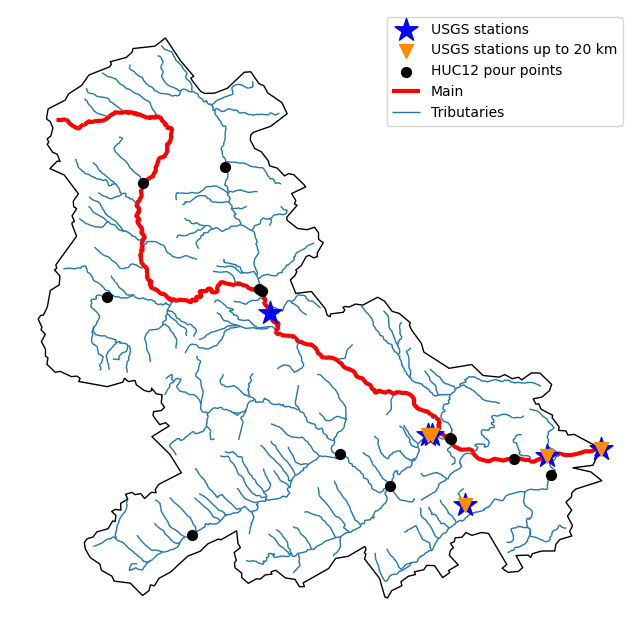Also, we can get the slope data for each river segment from the NHDPlus VAA database:

```vaa = nhd.nhdplus_vaa("input_data/nhdplus_vaa.parquet")

flw_trib["comid"] = pd.to_numeric(flw_trib.nhdplus_comid)
slope = gpd.GeoDataFrame(
pd.merge(flw_trib, vaa[["comid", "slope"]], left_on="comid", right_on="comid"),
crs=flw_trib.crs,
)
slope[slope.slope < 0] = np.nan
```

Additionally, we can obtain cross-section lines along the main river channel with 4 km spacing and width of 2 km using `network_xsection` as follows:

```from pynhd import NHD

distance = 4000  # in meters
width = 2000  # in meters
nhd = NHD("flowline_mr")
main_nhd = nhd.byids("COMID", flw_main.index)
main_nhd = pynhd.prepare_nhdplus(main_nhd, 0, 0, 0, purge_non_dendritic=True)
main_nhd = main_nhd.to_crs("ESRI:102003")
cs = pynhd.network_xsection(main_nhd, distance, width)
```

Then, we can use Py3DEP to obtain the elevation profile along the cross-section lines.

Now, let’s explore the PyGeoAPI capabilities. There are two ways that you can access PyGeoAPI: `PyGeoAPI` class and `pygeoapi` function. The `PyGeoAPI` class is for querying the database for a single location using tuples and list while the `pygeoapi` function is for querying the database for multiple locations at once and accepts a `geopandas.GeoDataFrame` as input. The `pygeoapi` function is more efficient than the `PyGeoAPI` class and has a simpler interface. In future versions, the `PyGeoAPI` class will be deprecated and the `pygeoapi` function will be the only way to access the database. Let’s compare the two, starting by `PyGeoAPI`:

```pygeoapi = PyGeoAPI()

trace = pygeoapi.flow_trace((1774209.63, 856381.68), crs="ESRI:102003", direction="none")

split = pygeoapi.split_catchment((-73.82705, 43.29139), crs="epsg:4326", upstream=False)

profile = pygeoapi.elevation_profile(
[(-103.801086, 40.26772), (-103.80097, 40.270568)],
numpts=101,
dem_res=1,
crs="epsg:4326",
)

section = pygeoapi.cross_section((-103.80119, 40.2684), width=1000.0, numpts=101, crs="epsg:4326")
```

Now, let’s do the same operations using `pygeoapi`:

```import geopandas as gpd
import shapely.geometry as sgeom
import pynhd as nhd

coords = gpd.GeoDataFrame(
{
"direction": ["up", "down"],
"upstream": [True, False],
"width": [1000.0, 500.0],
"numpts": [101, 55],
},
geometry=[
sgeom.Point(-73.82705, 43.29139),
sgeom.Point(-103.801086, 40.26772),
],
crs="epsg:4326",
)
trace = nhd.pygeoapi(coords, "flow_trace")
split = nhd.pygeoapi(coords, "split_catchment")
section = nhd.pygeoapi(coords, "cross_section")

coords = gpd.GeoDataFrame(
{
"direction": ["up", "down"],
"upstream": [True, False],
"width": [1000.0, 500.0],
"numpts": [101, 55],
"dem_res": [1, 10],
},
geometry=[
sgeom.MultiPoint([(-103.801086, 40.26772), (-103.80097, 40.270568)]),
sgeom.MultiPoint([(-102.801086, 39.26772), (-102.80097, 39.270568)]),
],
crs="epsg:4326",
)
profile = nhd.pygeoapi(coords, "elevation_profile")
```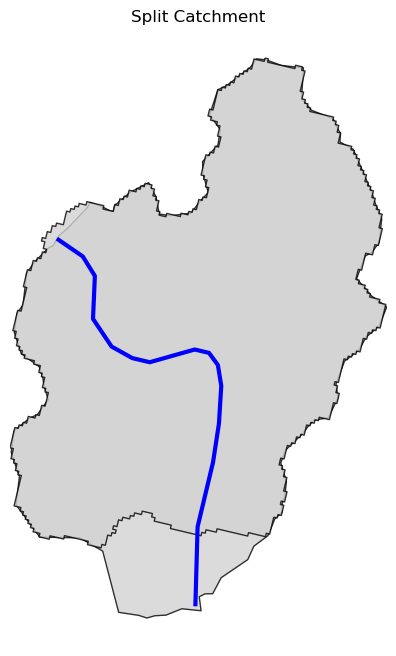Next, we retrieve mid- and high-resolution flowlines within the bounding box of our watershed and compare them using `WaterData` for mid-resolution, `NHDPlusHR` for high-resolution.

```mr = WaterData("nhdflowline_network")
nhdp_mr = mr.bybox(basin.geometry.bounds)

hr = NHDPlusHR("flowline")
nhdp_hr = hr.bygeom(basin.geometry.bounds)
```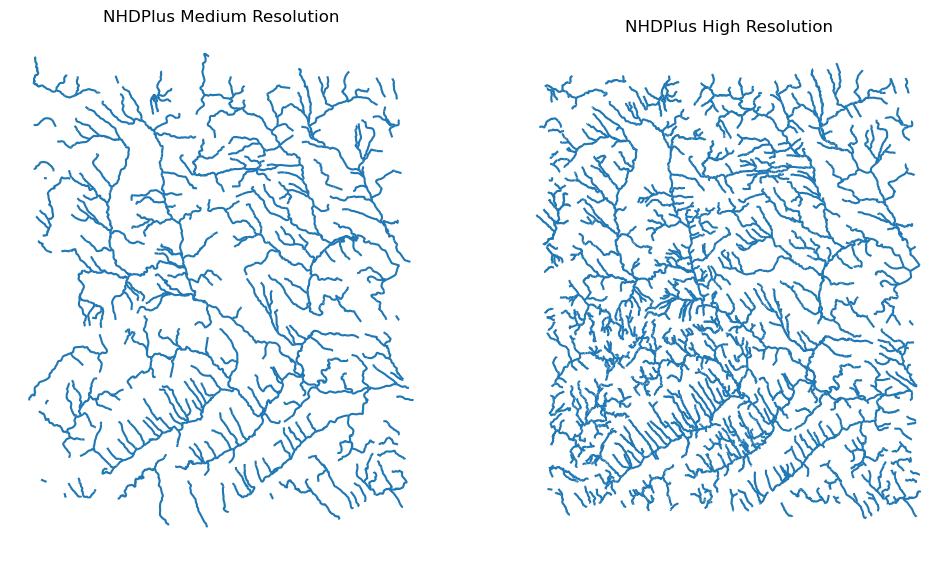An alternative to `WaterData` and `NHDPlusHR` is the `NHD` class that supports both the mid- and high-resolution NHDPlus V2 data:

```mr = NHD("flowline_mr")
nhdp_mr = mr.bygeom(basin.geometry.bounds)

hr = NHD("flowline_hr")
nhdp_hr = hr.bygeom(basin.geometry.bounds)
```

Moreover, `WaterData` can find features within a given radius (in meters) of a point:

```eck4 = "+proj=eck4 +lon_0=0 +x_0=0 +y_0=0 +datum=WGS84 +units=m +no_defs"
coords = (-5727797.427596455, 5584066.49330473)
```

Instead of getting all features within a radius of the coordinate, we can snap to the closest feature ID using NLDI:

```comid_closest = nldi.comid_byloc((x, y), eck4)
flw_closest = nhdp_mr.byid("comid", comid_closest.comid.values)
```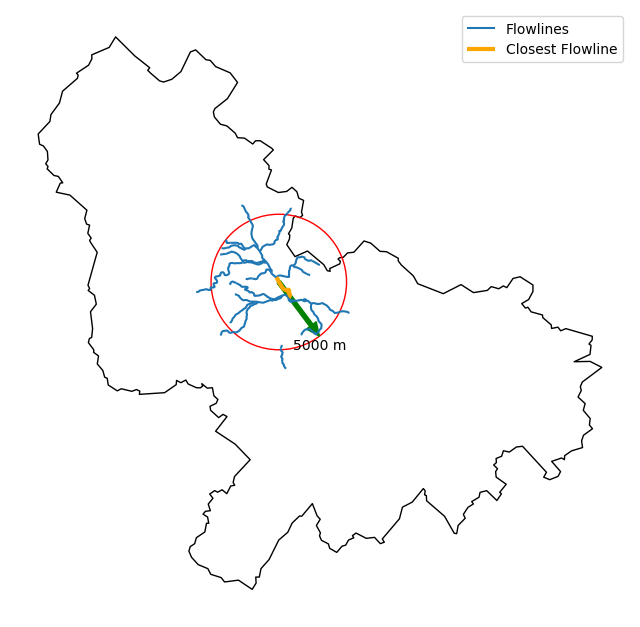Since NHDPlus HR is still at the pre-release stage let’s use the MR flowlines to demonstrate the vector-based accumulation. Based on a topological sorted river network `pynhd.vector_accumulation` computes flow accumulation in the network. It returns a data frame that is sorted from upstream to downstream that shows the accumulated flow in each node.

PyNHD has a utility called `prepare_nhdplus` that identifies such relationships among other things such as fixing some common issues with NHDPlus flowlines. But first, we need to get all the NHDPlus attributes for each ComID since `NLDI` only provides the flowlines’ geometries and ComIDs which is useful for navigating the vector river network data. For getting the NHDPlus database we use `WaterData`. Let’s use the `nhdflowline_network` layer to get required info.

```wd = WaterData("nhdflowline_network")

comids = flw_trib.nhdplus_comid.to_list()
nhdp_trib = wd.byid("comid", comids)
flw = nhd.prepare_nhdplus(nhdp_trib, 0, 0, purge_non_dendritic=False)
```

To demonstrate the use of routing, let’s use `nhdplus_attrs` function to get a list of available NHDPlus attributes

```char = "CAT_RECHG"
area = "areasqkm"

local = nldi.getcharacteristic_byid(comids, "local", char_ids=char)
flw = flw.merge(local[char], left_on="comid", right_index=True)

def runoff_acc(qin, q, a):
return qin + q * a

flw_r = flw[["comid", "tocomid", char, area]]
runoff = nhd.vector_accumulation(flw_r, runoff_acc, char, [char, area])

def area_acc(ain, a):
return ain + a

flw_a = flw[["comid", "tocomid", area]]
areasqkm = nhd.vector_accumulation(flw_a, area_acc, area, [area])

runoff /= areasqkm
```

Since these are catchment-scale characteristics, let’s get the catchments then add the accumulated characteristic as a new column and plot the results.

```wd = WaterData("catchmentsp")
catchments = wd.byid("featureid", comids)

c_local = catchments.merge(local, left_on="featureid", right_index=True)
c_acc = catchments.merge(runoff, left_on="featureid", right_index=True)
```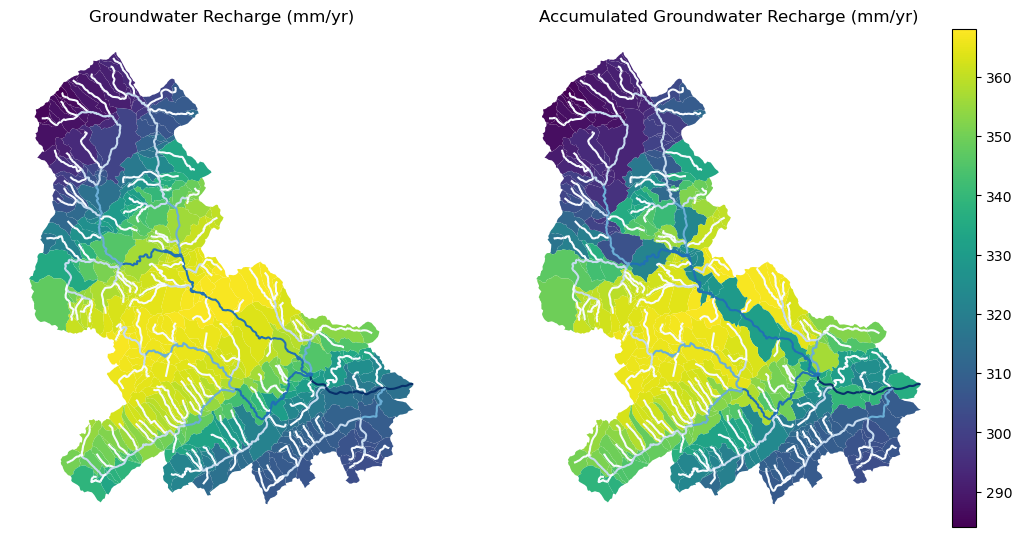More examples can be found here.